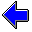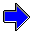Java Security Settings:
This web page employs Java, which requires specific security settings for correct operation.
If the applets on this page do not run correctly, consult the Virtual Chemistry Experiments FAQ
or the Physlet Physics web site for establishing the correct security settings.

# Gas Laws

## Ideal Gas Law and the Gas Constant

### Concepts

At this point, you have experimentally explored three gas laws.

Boyle's Law
For a constant amount of gas at a constant temperature, the product of the pressure and volume of the gas is a constant.

P V = constantBL

Charles's Law
For a constant amount of gas at a constant pressure, the volume of the gas is directly proportional to the absolute temperature.

V = constantCL T

At a given temperature and pressure, equal volumes of gas contain equal numbers of moles.

V = constantAL n

In each of these laws, the identity of the gas is unimportant.

Intuitively, one expects that each of these laws is a special case of a more general law. That general law is called the Ideal Gas Law.

P V = n R T

The constant R is called the gas constant, and its physical significance will be explored in subsequent experiments.

### Experiment

Objectives

• Verify the applicability of the ideal gas law.
• Determine the value of the gas constant.

The molar concentration, C, of a gas is defined to be C = n/V. Using this definition, the ideal gas law can be rewritten as

P = R T C

In effect, the pressure of the gas should be directly proportional to both the molar concentration and the temperature.

In the previous experiment, you saw how the molar concentration of a gas can be measured experimentally. This same technique is employed in this experiment to measure the molar concentration of nitrogen at various pressures and temperatures.

To perform the experiment:

1. Select a temperature
2. Add nitrogen gas to the bulb
3. Measure the pressure and mass of the gas
4. Calculate the molar concentration of the gas
5. Plot the pressure vs the molar concentration on the graph
6. Repeat Steps 2-5 at least five times, so that the graph contains at least five points
7. Examine the plot to determine if the ideal gas law is obeyed
8. Use the slope of the plot to determine the gas constant in units of L atm mole-1 K-1
9. Repeat the entire experiment at a different temperature. Do you obtain the same value for the gas constant?

The glass bulb has a volume of 2.00 L.

Temperature: oC

Pressure: torr

Concentration: mole/L

Concentration
( mole/L )
Pressure
( torr )

Slope = torr L/mole

Intercept = torrAvogadro's Law                     Dalton's LawGas Laws Home PageVirtual Chemistry Home Page

GasConstant.html version 2.1